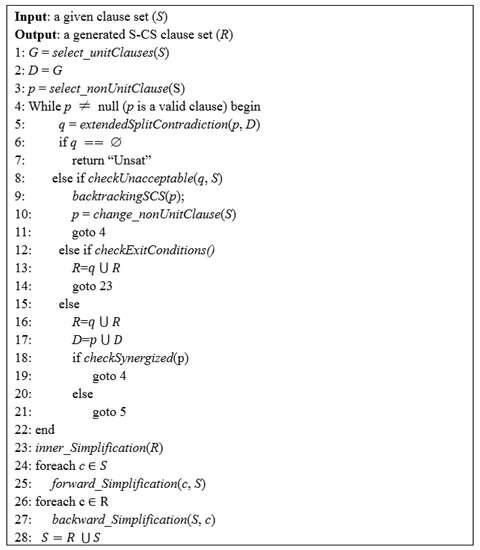Next Article in Journal
Visual Analysis of the Newton’s Method with Fractional Order Derivatives
Previous Article in Journal
A New Identity Involving Balancing Polynomials and Balancing Numbers
Open AccessArticle

# CSE_E 1.0: An Integrated Automated Theorem Prover for First-Order Logic

by 1,2,*, 2,3, 2,4, 2,3 and 1,2
1
School of Information Science and Technology, Southwest Jiaotong University, Chengdu 610031, China
2
National-Local Joint Engineering Lab of System Credibility Automatic Verification, Chengdu 610031, China
3
School of Mathematics, Southwest Jiaotong University, Chengdu 610031, China
4
School of Computing, Ulster University, Belfast BT37 0QB , Northern Ireland, UK
*
Author to whom correspondence should be addressed.
Symmetry 2019, 11(9), 1142; https://doi.org/10.3390/sym11091142
Received: 12 August 2019 / Revised: 29 August 2019 / Accepted: 6 September 2019 / Published: 8 September 2019
First-order logic is an important part of mathematical logic, and automated theorem proving is an interdisciplinary field of mathematics and computer science. The paper presents an automated theorem prover for first-order logic, called $C S E _ E$ 1.0, which is a combination of two provers contradiction separation extension (CSE) and E, where CSE is based on the recently-introduced multi-clause standard contradiction separation (S-CS) calculus for first-order logic and E is the well-known equational theorem prover for first-order logic based on superposition and rewriting. The motivation of the combined prover $C S E _ E$ 1.0 is to (1) evaluate the capability, applicability and generality of $C S E _ E$ , and (2) take advantage of novel multi-clause S-CS dynamic deduction of CSE and mature equality handling of E to solve more and harder problems. In contrast to other improvements of E, $C S E _ E$ 1.0 optimizes E mainly from the inference mechanism aspect. The focus of the present work is given to the description of $C S E _ E$ including its S-CS rule, heuristic strategies, and the S-CS dynamic deduction algorithm for implementation. In terms of combination, in order not to lose the capability of E and use $C S E _ E$ to solve some hard problems which are unsolved by E, $C S E _ E$ 1.0 schedules the running of the two provers in time. It runs plain E first, and if E does not find a proof, it runs plain CSE, then if it does not find a proof, some clauses inferred in the CSE run as lemmas are added to the original clause set and the combined clause set handed back to E for further proof search. $C S E _ E$ 1.0 is evaluated through benchmarks, e.g., CASC-26 (2017) and CASC-J9 (2018) competition problems (FOFdivision). Experimental results show that $C S E _ E$ 1.0 indeed enhances the performance of E to a certain extent. View Full-Text
Show FiguresFigure 1

MDPI and ACS Style

Cao, F.; Xu, Y.; Liu, J.; Chen, S.; Ning, X. CSE_E 1.0: An Integrated Automated Theorem Prover for First-Order Logic. Symmetry 2019, 11, 1142.

Show more citation formats Show less citations formats
Note that from the first issue of 2016, MDPI journals use article numbers instead of page numbers. See further details here.

1